# Compute source power estimate by projecting the covariance with MNE#

We can apply the MNE inverse operator to a covariance matrix to obtain an estimate of source power. This is computationally more efficient than first estimating the source timecourses and then computing their power. This code is based on the code from  and has been useful to correct for individual field spread using source localization in the context of predictive modeling.

## Compute empty-room covariance#

First we compute an empty-room covariance, which captures noise from the sensors and environment.

```raw_empty_room_fname = data_path / "MEG" / "sample" / "ernoise_raw.fif"
raw_empty_room.crop(0, 30)  # cropped just for speed
noise_cov = mne.compute_raw_covariance(raw_empty_room, method="shrunk")
del raw_empty_room
```
```Opening raw data file /home/circleci/mne_data/MNE-sample-data/MEG/sample/ernoise_raw.fif...
Read a total of 3 projection items:
PCA-v1 (1 x 102)  idle
PCA-v2 (1 x 102)  idle
PCA-v3 (1 x 102)  idle
Range : 19800 ... 85867 =     32.966 ...   142.965 secs
4 projection items deactivated
Using up to 150 segments
Computing rank from data with rank=None
Using tolerance 2.6e-09 (2.2e-16 eps * 305 dim * 3.9e+04  max singular value)
Estimated rank (mag + grad): 302
MEG: rank 302 computed from 305 data channels with 3 projectors
Created an SSP operator (subspace dimension = 3)
Setting small MEG eigenvalues to zero (without PCA)
Reducing data rank from 305 -> 302
Estimating covariance using SHRUNK
Done.
Number of samples used : 18000
[done]
```

## Epoch the data#

```raw.pick(["meg", "stim", "eog"]).load_data().filter(4, 12)
events = mne.find_events(raw, stim_channel="STI 014")
event_id = dict(aud_l=1, aud_r=2, vis_l=3, vis_r=4)
tmin, tmax = -0.2, 0.5
baseline = (None, 0)  # means from the first instant to t = 0
epochs = mne.Epochs(
raw,
events,
event_id,
tmin,
tmax,
proj=True,
picks=("meg", "eog"),
baseline=None,
reject=reject,
decim=5,
verbose="error",
)
del raw
```
```Removing projector <Projection | Average EEG reference, active : False, n_channels : 60>
Reading 0 ... 41699  =      0.000 ...   277.709 secs...
Filtering raw data in 1 contiguous segment
Setting up band-pass filter from 4 - 12 Hz

FIR filter parameters
---------------------
Designing a one-pass, zero-phase, non-causal bandpass filter:
- Windowed time-domain design (firwin) method
- Hamming window with 0.0194 passband ripple and 53 dB stopband attenuation
- Lower passband edge: 4.00
- Lower transition bandwidth: 2.00 Hz (-6 dB cutoff frequency: 3.00 Hz)
- Upper passband edge: 12.00 Hz
- Upper transition bandwidth: 3.00 Hz (-6 dB cutoff frequency: 13.50 Hz)
- Filter length: 249 samples (1.658 s)

[Parallel(n_jobs=1)]: Done  17 tasks      | elapsed:    0.0s
[Parallel(n_jobs=1)]: Done  71 tasks      | elapsed:    0.1s
[Parallel(n_jobs=1)]: Done 161 tasks      | elapsed:    0.3s
[Parallel(n_jobs=1)]: Done 287 tasks      | elapsed:    0.5s
319 events found on stim channel STI 014
Event IDs: [ 1  2  3  4  5 32]
```

## Compute and plot covariances#

In addition to the empty-room covariance above, we compute two additional covariances:

1. Baseline covariance, which captures signals not of interest in our analysis (e.g., sensor noise, environmental noise, physiological artifacts, and also resting-state-like brain activity / “noise”).

2. Data covariance, which captures our activation of interest (in addition to noise sources).

```base_cov = mne.compute_covariance(
epochs, tmin=-0.2, tmax=0, method="shrunk", verbose=True
)
data_cov = mne.compute_covariance(
epochs, tmin=0.0, tmax=0.2, method="shrunk", verbose=True
)

fig_noise_cov = mne.viz.plot_cov(noise_cov, epochs.info, show_svd=False)
fig_base_cov = mne.viz.plot_cov(base_cov, epochs.info, show_svd=False)
fig_data_cov = mne.viz.plot_cov(data_cov, epochs.info, show_svd=False)
```
•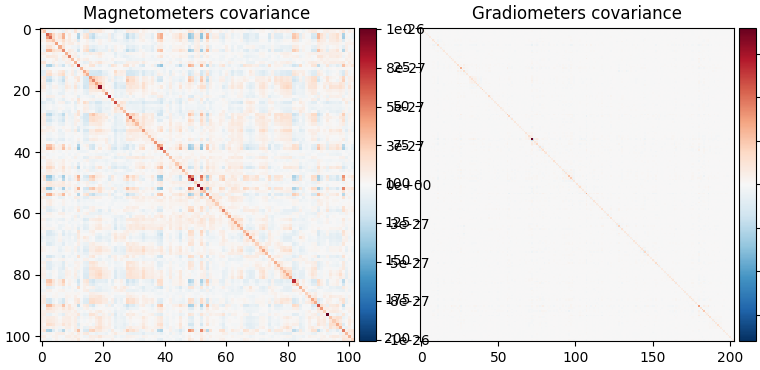•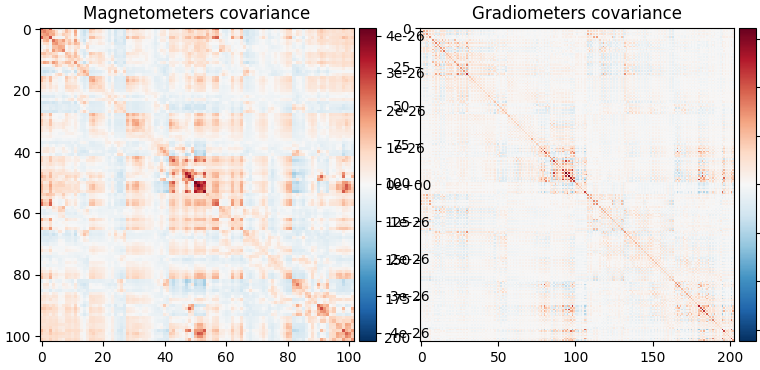•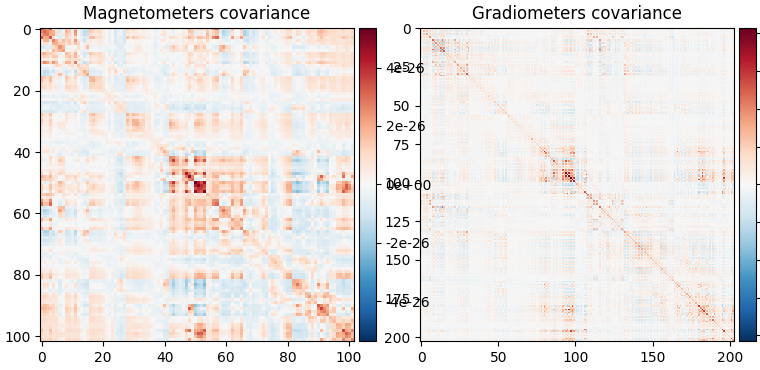```Computing rank from data with rank=None
Using tolerance 1.9e-09 (2.2e-16 eps * 305 dim * 2.9e+04  max singular value)
Estimated rank (mag + grad): 302
MEG: rank 302 computed from 305 data channels with 3 projectors
Created an SSP operator (subspace dimension = 3)
Setting small MEG eigenvalues to zero (without PCA)
Reducing data rank from 305 -> 302
Estimating covariance using SHRUNK
Done.
Number of samples used : 1680
[done]
Computing rank from data with rank=None
Using tolerance 2.2e-09 (2.2e-16 eps * 305 dim * 3.2e+04  max singular value)
Estimated rank (mag + grad): 302
MEG: rank 302 computed from 305 data channels with 3 projectors
Created an SSP operator (subspace dimension = 3)
Setting small MEG eigenvalues to zero (without PCA)
Reducing data rank from 305 -> 302
Estimating covariance using SHRUNK
Done.
Number of samples used : 1680
[done]
```

We can also look at the covariances using topomaps, here we just show the baseline and data covariances, followed by the data covariance whitened by the baseline covariance:

```evoked = epochs.average().pick("meg")
evoked.plot(time_unit="s")
evoked.plot_topomap(times=np.linspace(0.05, 0.15, 5), ch_type="mag")

loop = {
"Noise": (noise_cov, dict()),
"Data": (data_cov, dict()),
"Whitened data": (data_cov, dict(noise_cov=noise_cov)),
}
for title, (_cov, _kw) in loop.items():
fig.suptitle(title)
```
•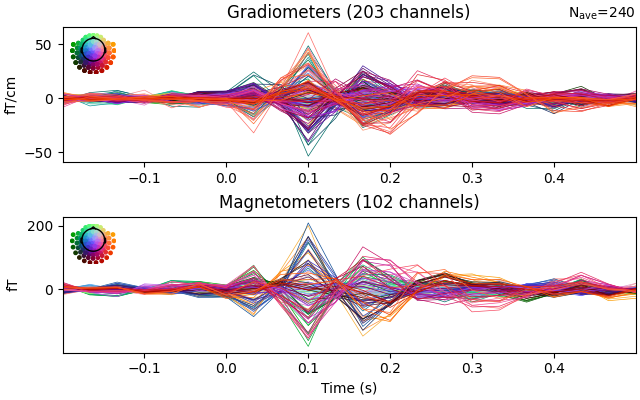•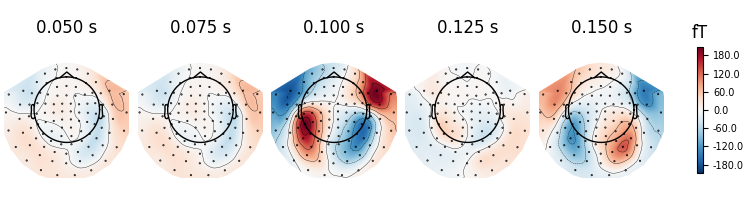•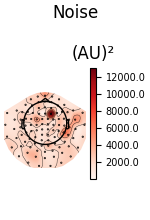•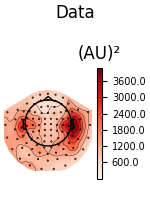•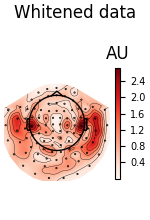```    Created an SSP operator (subspace dimension = 3)
Computing rank from covariance with rank=None
Using tolerance 1.4e-13 (2.2e-16 eps * 305 dim * 2.1  max singular value)
Estimated rank (mag + grad): 302
MEG: rank 302 computed from 305 data channels with 3 projectors
Setting small MEG eigenvalues to zero (without PCA)
Created the whitener using a noise covariance matrix with rank 302 (3 small eigenvalues omitted)
Created an SSP operator (subspace dimension = 3)
Computing rank from covariance with rank=None
Using tolerance 1.4e-13 (2.2e-16 eps * 305 dim * 2.1  max singular value)
Estimated rank (mag + grad): 302
MEG: rank 302 computed from 305 data channels with 3 projectors
Setting small MEG eigenvalues to zero (without PCA)
Created the whitener using a noise covariance matrix with rank 302 (3 small eigenvalues omitted)
```

## Apply inverse operator to covariance#

Finally, we can construct an inverse using the empty-room noise covariance:

```# Read the forward solution and compute the inverse operator
fname_fwd = meg_path / "sample_audvis-meg-oct-6-fwd.fif"
# make an MEG inverse operator
info = evoked.info
inverse_operator = make_inverse_operator(info, fwd, noise_cov, loose=0.2, depth=0.8)
```
```Reading forward solution from /home/circleci/mne_data/MNE-sample-data/MEG/sample/sample_audvis-meg-oct-6-fwd.fif...
Computing patch statistics...
[done]
Computing patch statistics...
[done]
Desired named matrix (kind = 3523) not available
Read MEG forward solution (7498 sources, 306 channels, free orientations)
Source spaces transformed to the forward solution coordinate frame
Converting forward solution to surface orientation
Average patch normals will be employed in the rotation to the local surface coordinates....
Converting to surface-based source orientations...
[done]
Computing inverse operator with 305 channels.
305 out of 306 channels remain after picking
Selected 305 channels
Creating the depth weighting matrix...
203 planar channels
limit = 7265/7498 = 10.037795
scale = 2.52065e-08 exp = 0.8
Applying loose dipole orientations to surface source spaces: 0.2
Whitening the forward solution.
Created an SSP operator (subspace dimension = 3)
Computing rank from covariance with rank=None
Using tolerance 1.4e-13 (2.2e-16 eps * 305 dim * 2.1  max singular value)
Estimated rank (mag + grad): 302
MEG: rank 302 computed from 305 data channels with 3 projectors
Setting small MEG eigenvalues to zero (without PCA)
Creating the source covariance matrix
Computing SVD of whitened and weighted lead field matrix.
largest singular value = 6.40105
scaling factor to adjust the trace = 5.62956e+19 (nchan = 305 nzero = 3)
```

Project our data and baseline covariance to source space:

```stc_data = apply_inverse_cov(
data_cov,
evoked.info,
inverse_operator,
nave=len(epochs),
method="dSPM",
verbose=True,
)
stc_base = apply_inverse_cov(
base_cov,
evoked.info,
inverse_operator,
nave=len(epochs),
method="dSPM",
verbose=True,
)
```
```Preparing the inverse operator for use...
Scaled noise and source covariance from nave = 1 to nave = 240
Created the regularized inverter
Created an SSP operator (subspace dimension = 3)
Created the whitener using a noise covariance matrix with rank 302 (3 small eigenvalues omitted)
Computing noise-normalization factors (dSPM)...
[done]
Applying inverse operator to "cov"...
Picked 305 channels from the data
Computing inverse...
Eigenleads need to be weighted ...
Computing residual...
Explained  37.2% variance
dSPM...
Combining the current components...
[done]
Preparing the inverse operator for use...
Scaled noise and source covariance from nave = 1 to nave = 240
Created the regularized inverter
Created an SSP operator (subspace dimension = 3)
Created the whitener using a noise covariance matrix with rank 302 (3 small eigenvalues omitted)
Computing noise-normalization factors (dSPM)...
[done]
Applying inverse operator to "cov"...
Picked 305 channels from the data
Computing inverse...
Eigenleads need to be weighted ...
Computing residual...
Explained  37.2% variance
dSPM...
Combining the current components...
[done]
```

And visualize power is relative to the baseline:

```stc_data /= stc_base
brain = stc_data.plot(
subject="sample",
subjects_dir=subjects_dir,
clim=dict(kind="percent", lims=(50, 90, 98)),
smoothing_steps=7,
)
```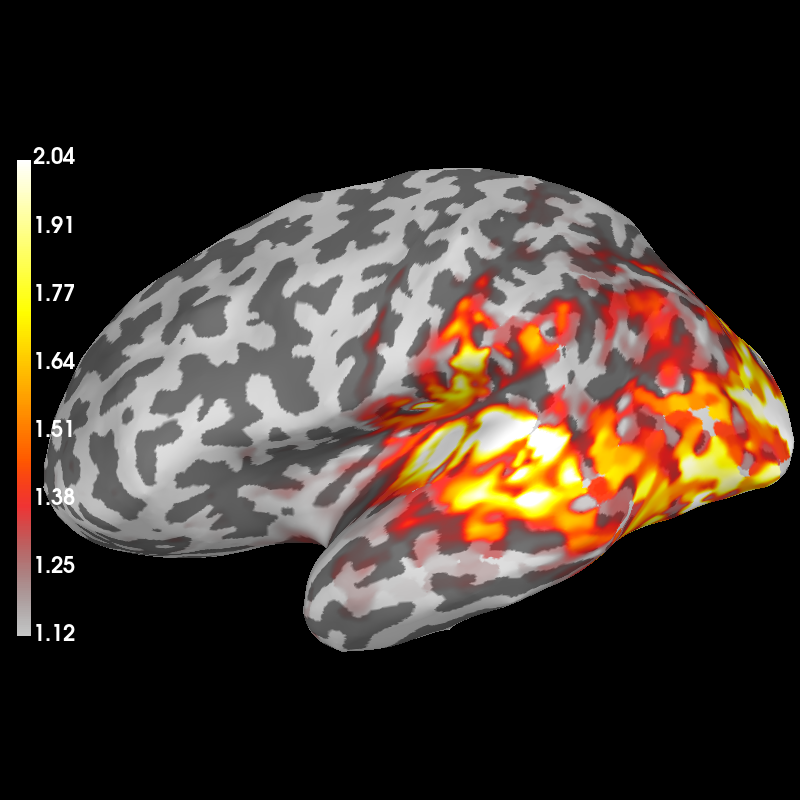```Using control points [1.11870454 1.45629497 2.03742168]
```

Total running time of the script: (0 minutes 28.102 seconds)

Estimated memory usage: 192 MB

Gallery generated by Sphinx-Gallery